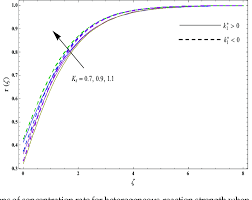## How to Calculate and Solve for Rate of Homogeneous Reaction | CorrosionThe image above represents rate of homogeneous reaction.

To compute for rate of homogeneous reaction, two essential parameters are needed and these parameters are dn/dt and Stoichiometric Number (v).

The formula for calculating rate of homogeneous reaction:

Vr = dn/dt / v

Where:

Vr = Rate of Homogeneous Reaction
dn/dt = dn/dt
v = Stoichiometric Number

Let’s solve an example;
Find the rate of homogeneous reaction when the dn/dt is 24 and the stoichiometric number is 12.

This implies that;

dn/dt = dn/dt = 24
v = Stoichiometric Number = 12

Vr = dn/dt / v
Vr = 24 / 12
Vr = 2

Therefore, the rate of homogeneous reaction is 2.

Calculating the dn/dt when the Rate of Homogeneous Reaction and the Stoichiometric Number is Given.

dn/dt = Vr x v

Where;

dn/dt = dn/dt
Vr = Rate of Homogeneous Reaction
v = Stoichiometric Number

Let’s solve an example;
Find the dn/dt when the rate of homogeneous reaction is 18 and the stoichiometric number is 4.

This implies that;

Vr = Rate of Homogeneous Reaction = 18
v = Stoichiometric Number = 4

dn/dt = Vr x v
dn/dt = 18 x 4
dn/dt = 72

Therefore, the dn/dt is 72.